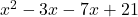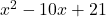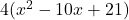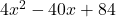## Which is the polynomial function of lowest degree with leading coefficient of 4 and roots 7 and 3? O f(x)= x2 – 3x-+3-17 O f(x)=

Question

Which is the polynomial function of lowest degree with leading coefficient of 4 and roots 7 and 3?
O f(x)= x2 – 3x-+3-17
O f(x)= 4x² -1245x+3/7
O f(x)=x2 – 3x? – X+21
O x) = 4x° – 12x – 28x+84

in progress 0
5 months 2021-08-23T09:34:46+00:00 1 Answers 0 views 0

## Answers ( )which simplifies toand we distribute in the 4:to getwhich is another way to write the last choice you’re given.